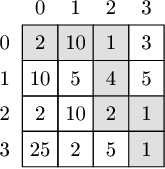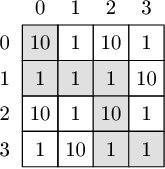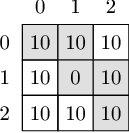Your browser (Unknown 0) is no longer supported. Some parts of the website may not work correctly. Please update your browser.

#### MinTrailingZeros

Find a path in given matrix, such that the product of all the numbers on the path has the minimal number of trailing zeros.

Matrix A, consisting of N rows and N columns of non-negative integers, is given. Rows are numbered from 0 to N−1 (from top to bottom). Columns are numbered from 0 to N−1 (from left to right). We would like to find a path that starts at the upper-left corner (0, 0) and, moving only right and down, finishes at the bottom-right corner (N−1, N−1). Then, all the numbers on this path will be multiplied together.

Find a path such that the product of all the numbers on the path contain the minimal number of trailing zeros. We assume that 0 has 1 trailing zero.

Write a function:

class Solution { public int solution(int[][] A); }

that, given matrix A, returns the minimal number of trailing zeros.

Examples:

1. Given matrix A below:the function should return 1. The optimal path is: (0,0) → (0,1) → (0,2) → (1,2) → (2,2) → (2,3) → (3,3). The product of numbers 2, 10, 1, 4, 2, 1, 1 is 160, which has one trailing zero. There is no path that yields a product with no trailing zeros.

2. Given matrix A below:the function should return 2. One of the optimal paths is: (0,0) → (1,0) → (1,1) → (1,2) → (2,2) → (3,2) → (3,3). The product of numbers 10, 1, 1, 1, 10, 1, 1 is 100, which has two trailing zeros. There is no path that yields a product with fewer than two trailing zeros.

3. Given matrix A below:the function should return 1. One of the optimal paths is: (0,0) → (0,1) → (1,1) → (1,2) → (2,2). The product of numbers 10, 10, 0, 10, 10 is 0, which has one trailing zero. There is no path that yields a product with no trailing zeros.

Write an efficient algorithm for the following assumptions:

• N is an integer within the range [1..500];
• each element of matrix A is an integer within the range [0..1,000,000,000].